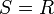# Normal domain

This article defines a property of integral domains, viz., a property that, given any integral domain, is either true or false for that.
View other properties of integral domains | View all properties of commutative unital rings
VIEW RELATED: Commutative unital ring property implications | Commutative unital ring property non-implications |Commutative unital ring metaproperty satisfactions | Commutative unital ring metaproperty dissatisfactions | Commutative unital ring property satisfactions | Commutative unital ring property dissatisfactions

## Definition

An integral domain$R$ is said to be normal if it satisfies the following equivalent conditions:

1.$R$ is integrally closed in its field of fractions.
2. If$S$ is a subring of the field of fractions of$R$ that contains$R$ as a subgroup of finite index, then$S = R$.

### Equivalence of definitions

Further information: equivalence of definitions of normal domain

## Relation with other properties

### Stronger properties

Property Meaning Proof of implication Proof of strictness (reverse implication failure) Intermediate notions
unique factorization domain integral domain with unique factorization unique factorization implies normal normal domain not implies UFD Template:Intermediate notion short
principal ideal domain integral domain where every ideal is principal (via UFD) (via UFD) click here
Euclidean domain integral domain having a Euclidean norm (via UFD) (via UFD) click here
Noetherian normal domain normal domain that is also a Noetherian ring click here

### Weaker properties

Property Meaning Proof of implication Proof of strictness (reverse implication failure) Intermediate notions

## Metaproperties

### Closure under taking localizations

This property of integral domains is closed under taking localizations: the localization at a multiplicatively closed subset of a commutative unital ring with this property, also has this property. In particular, the localization at a prime ideal, and the localization at a maximal ideal, have the property.
View other localization-closed properties of integral domains | View other localization-closed properties of commutative unital rings

In fact, the localization at a multiplicatively closed subset of a normal domain continues to be a normal domain. For full proof, refer: Normality is localization-closed

### Polynomial-closedness

This property of integral domains is closed under taking polynomials, i.e., whenever an integral domain has this property, so does the polynomial ring in one variable over it.
View other polynomial-closed properties of integral domains OR view polynomial-closed properties of commutative unital rings

The polynomial ring in one variable over a normal domain is again normal. For full proof, refer: Normality is polynomial-closed

### Ring of integer-valued polynomials

The ring of integer-valued polynomials over a normal domain is again a normal domain. For full proof, refer: Ring of integer-valued polynomials over normal domain is normal